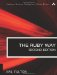# Section 5.5. Formatting Numbers for Output

### 5.4. Comparing Floating Point Numbers

It is a sad fact of life that computers do not represent floating point values exactly. The following code fragment, in a perfect world, would print "yes"; on every architecture we have tried, it will print "no" instead:

`x = 1000001.0/0.003 y = 0.003*x if y == 1000001.0   puts "yes" else   puts "no" end`

The reason, of course, is that a floating point number is stored in some finite number of bits; and no finite number of bits is adequate to store a repeating decimal with an infinite number of digits.

Because of this inherent inaccuracy in floating point comparisons, we may find ourselves in situations (like the one we just saw) in which the values we are comparing are the same for all practical purposes, but the hardware stubbornly thinks they are different.

The following code is a simple way to ensure that floating point comparisons are done "with a fudge factor"that is, the comparisons will be done within any tolerance specified by the programmer:

`class Float   EPSILON = 1e-6   # 0.000001   def ==(x)     (self-x).abs < EPSILON   end end x = 1000001.0/0.003 y = 0.003*x if y == 1.0         # Using the new ==   puts "yes"        # Now we output "yes" else   puts "no" end`

We may find that we want different tolerances for different situations. For this case, we define a new method equals? as a member of Float. (This name avoids confusion with the standard methods equal? and eql?; the latter in particular should not be overridden.)

`class Float   EPSILON = 1e-6   def equals?(x, tolerance=EPSILON)     (self-x).abs < tolerance   end end flag1 = (3.1416).equals? Math::PI          # false flag2 = (3.1416).equals?(Math::PI, 0.001)  # true`

We could also use a different operator entirely to represent approximate equality; the =~ operator might be a good choice.

Bear in mind that this sort of thing is not a real solution. As successive computations are performed, error is compounded. If you must use floating point math, be prepared for the inaccuracies. If the inaccuracies are not acceptable, use BigDecimal or some other solution. (See section 5.8 "Using BigDecimal" and section 5.9 "Working with Rational Values.")The Ruby Way, Second Edition: Solutions and Techniques in Ruby Programming (2nd Edition)
ISBN: 0672328844
EAN: 2147483647
Year: 2004
Pages: 269
Authors: Hal Fulton

Similar book on Amazon

flylib.com © 2008-2017.
If you may any questions please contact us: flylib@qtcs.net